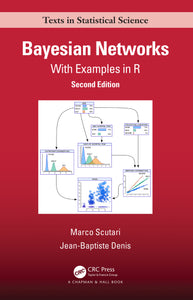# Bayesian Networks : With Examples in R

Regular price \$99.95 \$99.95 Sale

Author(s): Marco Scutari, Jean-Baptiste Denis

Bayesian Networks: With Examples in R, Second Edition introduces Bayesian networks using a hands-on approach. Simple yet meaningful examples illustrate each step of the modelling process and discuss side-by-side the underlying theory and its application using R code. The examples start from the simplest notions and gradually increase in complexity. In particular, this new edition contains significant new material on topics from modern machine learning practice: dynamic networks, networks with heterogeneous variables, and model validation.

The first three chapters explain the whole process of Bayesian network modelling, from structure learning to parameter learning to inference. These chapters cover discrete, Gaussian, and conditional Gaussian Bayesian networks. The following two chapters delve into dynamic networks (to model temporal data) and into networks including arbitrary random variables (using Stan). The book then gives a concise but rigorous treatment of the fundamentals of Bayesian networks and offers an introduction to causal Bayesian networks. It also presents an overview of R packages and other software implementing Bayesian networks. The final chapter evaluates two real-world examples: a landmark causal protein-signaling network published in Science and a probabilistic graphical model for predicting the composition of different body parts.

Covering theoretical and practical aspects of Bayesian networks, this book provides you with an introductory overview of the field. It gives you a clear, practical understanding of the key points behind this modelling approach and, at the same time, it makes you familiar with the most relevant packages used to implement real-world analyses in R. The examples covered in the book span several application fields, data-driven models and expert systems, probabilistic and causal perspectives, thus giving you a starting point to work in a variety of scenarios.

Online supplementary materials include the data sets and the code used in the book, which will all be made available from https://www.bnlearn.com/book-crc-2ed/

Review(s):

EAN: 9781000410389